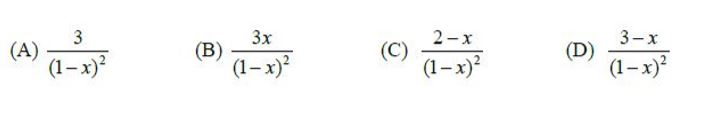# GATE | GATE CS 2018 | Question 18

• Last Updated : 09 Mar, 2018

Which one the following is a closed form expression for the generating function of the sequence {an}, where an = 2n + 3 for all n = 0, 1, 2,…?(A) A
(B) B
(C) C
(D) D

Explanation: Given an = 2n + 3

Generating function G(x) for the sequence an is
G(x) ==== 2(0+x+2x2+3x3+…..) + 3(1+x+x2+….)

We know that= 1+x+x2+….

x+2x2+3x3+… = x(1+2x+3x2+….) =Substituting calculated values in G(x)

G(x) ===Quiz of this Question

My Personal Notes arrow_drop_up